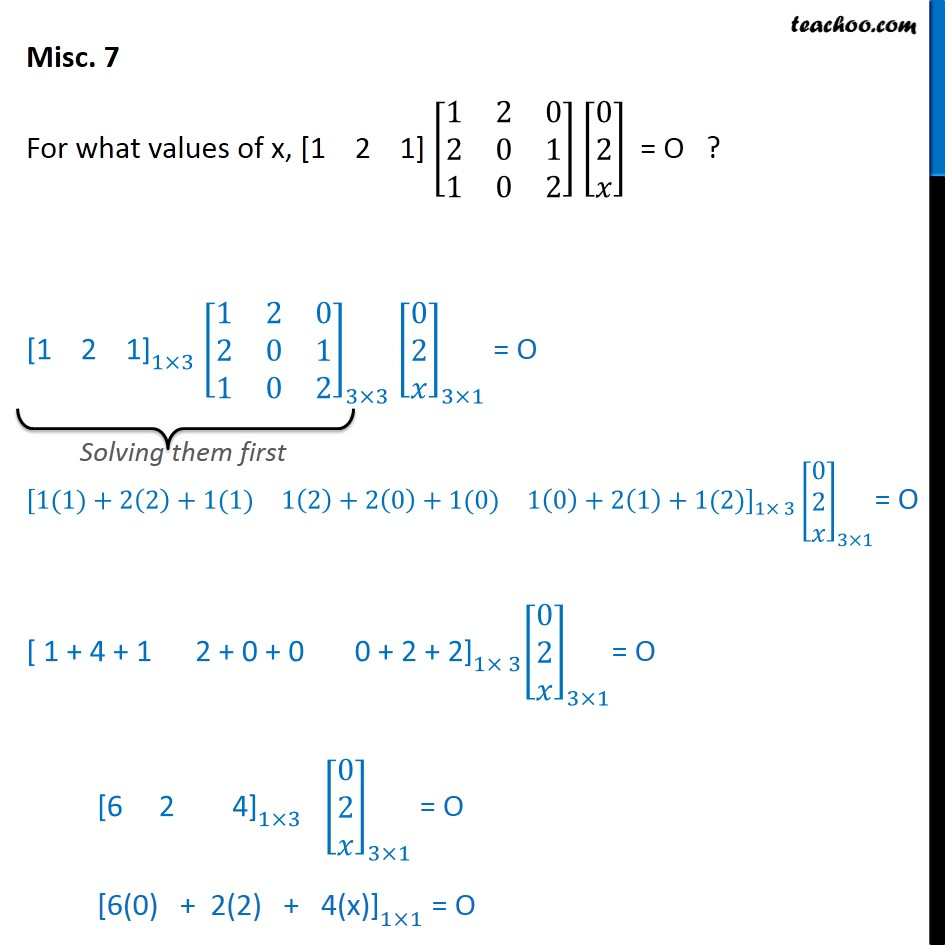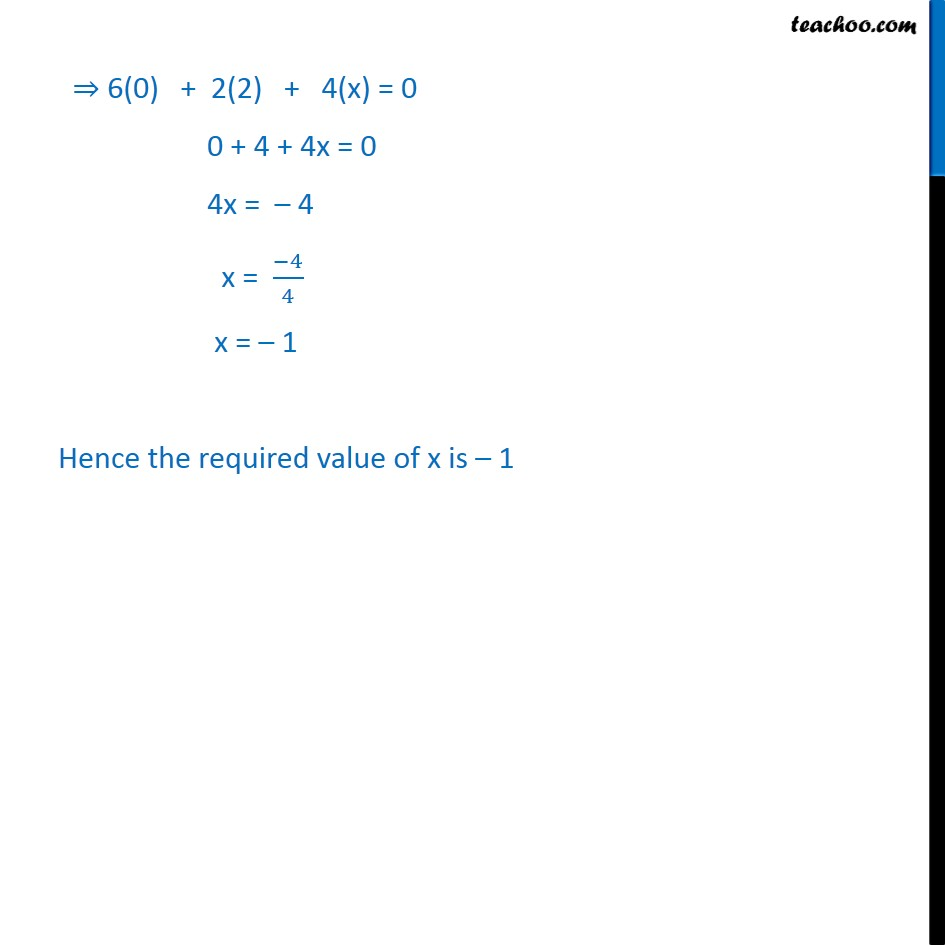Miscellaneous

Chapter 3 Class 12 Matrices
Serial order wiseMaths Crash Course - Live lectures + all videos + Real time Doubt solving!

### Transcript

Misc. 7 For what values of x, [1 2 1] [ 8(1&2&[email protected]&0&[email protected]&0&2)] [ 8([email protected]@ )] = O ? "[1 2 1]" _(1 3) [ 8(1&2&[email protected]&0&[email protected]&0&2)]_(3 3) [ 8([email protected]@ )]_(3 1) = O [ 8(1(1)+2(2)+1(1)&1(2)+2(0)+1(0)&1(0)+2(1)+1(2))]_(1 3) [ 8([email protected]@ )]_(3 1)= O "[ 1 + 4 + 1 2 + 0 + 0 0 + 2 + 2]" _(1 3) [ 8([email protected]@ )]_(3 1)= O "[6 2 4]" _(1 3) [ 8([email protected]@ )]_(3 1) = O "[6(0) + 2(2) + 4(x)]" _(1 1) = O "6(0) + 2(2) + 4(x)" = 0 0 + 4 + 4x = 0 4x = 4 x = ( 4)/4 x = 1 Hence the required value of x is 1